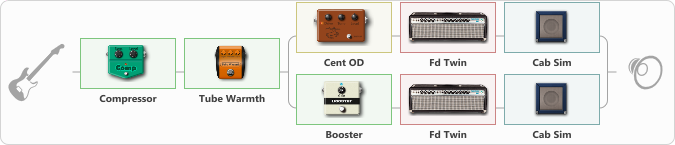# FendeR CruncH

Discussion in 'ToneLib-GFX presets' started by jackwhite, May 27, 2022.

1. FendeR CruncH

Preset name: FendeR CruncH

Bridge single Duo-Sonic

Effects chain:Effect: "Compressor" (Dynamics / Filter), active - "yes"
{
"Sense" = 52
"Level" = 22
}

Effect: "Tube Warmth" (Dynamics / Filter), active - "yes"
{
"Input" = 27
"Warmth" = 100
"Level" = 100
}

Effect: "Splitter" (Dynamics / Filter)
{
"A-Bypass" = Off
"A-Pan" = -25
"A-Level" = 55
"B-Bypass" = Off
"B-Pan" = 25
"B-Level" = 55
"Width" = 0

'A' branch:
{

Effect: "Cent OD" (Overdrive / Distortion), active - "yes"
{
"Drive" = 39
"Tone" = 100
"Level" = 99
}

Effect: "Fd Twin" (Amp simulators), active - "yes"
{
"Gain" = 47
"Bass" = 51
"Middle" = 50
"Treble" = 77
"Presence" = 50
"Master" = 50
"Level (dB)" = 0
}

Effect: "Cab Sim" (Cabinets), active - "yes"
{
"Model" = 2x12" Blackface
"Level (dB)" = 0
}
}
'B' branch:
{

Effect: "Booster" (Dynamics / Filter), active - "yes"
{
"Gain" = 100
}

Effect: "Fd Twin" (Amp simulators), active - "yes"
{
"Gain" = 47
"Bass" = 51
"Middle" = 50
"Treble" = 50
"Presence" = 50
"Master" = 50
"Level (dB)" = 0
}

Effect: "Cab Sim" (Cabinets), active - "yes"
{
"Model" = 2x12" Blackface
"Level (dB)" = 0
}
}
}

Note: You will need to download and install the ToneLib-GFX software to use the preset.

File size:
805 bytes
Views:
1,908